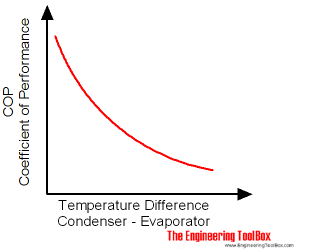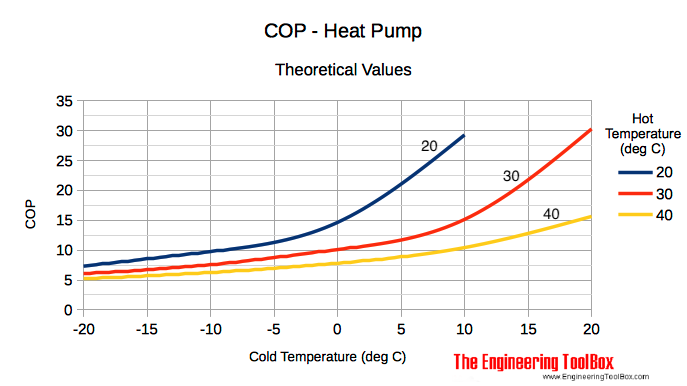Engineering ToolBox - Resources, Tools and Basic Information for Engineering and Design of Technical Applications!

# Heat Pumps - Performance and Efficiency Ratings

## Performance and efficiency rating of heat pumps.

Several methods can be used to rate heat pump performance and efficiency:

### COP - Coefficient of Performance

The Coefficient of Performance - COP - is the ratio of heat output to the amount of energy input for a heat pump.COP can be expressed as

COP = hh / hw                                            (1)

where

COP = Coefficient of Performance

hh = heat produced (Btu/h, J, kWh)

hw = equivalent electric energy input (Btu/h, J, kWh) = 3413 Pw

where

Pw = electrical input energy (W)

If a heat pump delivers 3 units of heat for every unit of energy input - the COP is 3.

• 1 kW = 1000 W = 3413 Btu/h

#### Example - COP Heat Pump

##### Cooling Cycle

A heat pump deliver 60000 Btu/h with a total electric input of 9 kW:

COP = (60000 Btu/h) / (3413 (9 kW))

= 1.95

##### Heating Cycle

A heat pump deliver 50000 Btu/h with a total input of 7 kW:

COP = (50000 Btu/h) / (3413 (7 kW))

= 2.1

#### Maximum COP

Maximum theoretical efficiency for a heating process is

COPheating = Th / (Th - Tc)                                               (1b)

COPheating = Coefficient of Performance - heating process

Th = absolute temperature on the hot side (K)

Tc = absolute temperature on the cold side (K)

Maximum theoretical efficiency for a cooling process is

COPcooling = Tc / (Th - Tc)                                             (1c)

COPcooling = Coefficient of Performance - cooling process

Note! - the efficiency of a cooling or heating process can be increased by reducing the temperature difference (Th - Tc) between the hot and cold side.

A heating process with a lower hot temperature - like achievable in a piped floor system - will increases the efficiency compared to a system with higher hot temperature - like a heating panel system. The opposite for a cooling process - a lower cold temperature will increase the efficiency.#### Example - Maximum Heat Pump Efficiency

An air to air heat pump operates between temperature -5 oC on the cold side and temperature 40 oC on the hot side. The maximum theoretical efficiency can be calculated by using (1b) as

COPheating = (40 + 273) / ((40 + 273) - (-5 + 273))

= 6.95

The typical practical value for a heat pump is in the range 2 - 4.

### EER - Energy Efficiency Ratio

The Energy Efficiency Ratio - EER - is a measure of the cooling efficiency of a heat pump.

EER can be expressed as

EER = hc / Pw                                                     (2)

where

EER = Energy Efficiency Rating

hc = cooling heat (Btu/h)

Pw = electrical power (W)

#### Example - EER

An air conditioner or heat pump in cooling mode draws 1000 W of electric power to produce 10000 Btu/h of cooling. The EER can be calculated as

EER = (10000 Btu/h) / (1000 W)

= 10

### HSPF - Heating Season Performance Factor

The Heating Season Performance Factor - HSPF - is a measure of the overall heating efficiency of a heat pump during the season.

HSPF = hs / 1000 Pws                                               (3)

where

hs = heat produced during the season (Btu)

Pws = electrical power consumed during the season (kWh)

The HSPF can be regarded as an "average" COP for an entire heating season. It is common to compare BTUs of heat output to watts of electrical energy input. HSPF of 6.8 can be compared with an average COP of 2. A HSPF in the range of 5-7 is acceptable.

#### Example - Heat Pump Heating Season Performance Factor

For a heat pump deliver 120,000,000 Btu during the season when consuming 15,000 kWh the HSPF can be calculated as

HSPF = (120000000 Btu) / (1000 (15000 kWh))

= 8

### SEER - Seasonal Energy Efficiency Ratio

Seasonal Energy Efficiency Ratio is a measure of the seasonal cooling efficiency of a heat pump or a consumer central air conditioning system.

The SEER should be at least 13 to be sold in the United States. SEER above 20 is a very efficient system.

## Related Topics

• ### Air Conditioning

Air Conditioning systems - heating, cooling and dehumidification of indoor air for thermal comfort.
• ### Heating

Heating systems - capacity and design of boilers, pipelines, heat exchangers, expansion systems and more.

## Related Documents

Calculating chiller and cooling tower refrigeration loads - in tons.
• ### Cooling and Heating - Performance and Efficiency Terminology

Performance and efficiency terminology related to heat pumps and air condition systems.
• ### Cooling Load - Convert between kW/ton to COP or EER

Convert between cooling load units like kW/ton, COP and EER.
• ### Efficiency

Efficiency is the ratio useful energy output to energy input.
• ### Steam Radiators and Convectors - Heating Capacities

Steam radiators and steam convectors - heating capacities and temperature coefficients.

## Engineering ToolBox - SketchUp Extension - Online 3D modeling!

Add standard and customized parametric components - like flange beams, lumbers, piping, stairs and more - to your Sketchup model with the Engineering ToolBox - SketchUp Extension - enabled for use with older versions of the amazing SketchUp Make and the newer "up to date" SketchUp Pro . Add the Engineering ToolBox extension to your SketchUp Make/Pro from the Extension Warehouse !

We don't collect information from our users. More about

## Citation

• The Engineering ToolBox (2008). Heat Pumps - Performance and Efficiency Ratings. [online] Available at: https://www.engineeringtoolbox.com/heat-pump-efficiency-ratings-d_1117.html [Accessed Day Month Year].

Modify the access date according your visit.

10.2.10